JMSLTM Numerical Library 7.2.0
com.imsl.datamining

## Class Apriori

• All Implemented Interfaces:
Serializable, Cloneable

```public class Apriori
extends Object
implements Serializable, Cloneable```
Performs the Apriori algorithm for association rule discovery.

Association rules are statements of the form, "if X, then Y", given with some measure of confidence. The main application for association rule discovery is market basket analysis, where X and Y are products or groups of products, and the occurrences are individual transactions, or "market baskets". The results help sellers learn relationships between the different products they sell, supporting better marketing decisions. There are other applications for association rule discovery, such as the problem areas of text mining and bioinformatics. The Apriori algorithm (Agrawal and Srikant, 1994) is one of the most popular algorithms for association rule discovery in transactional datasets.

In the first and most critical stage, the Apriori algorithm mines the transactions for frequent itemsets. An itemset is frequent if it appears in more than a minimum number of transactions. The number of transactions containing an itemset is known as its "support", and the minimum support (as a percentage of transactions) is a control parameter in the algorithm. The algorithm begins by finding the frequent single items. Then the algorithm generates all two-item sets from the frequent single items and determines which among them are frequent. From the collection of frequent pairs, Apriori forms candidate three-item subsets and determines which are frequent, and so on. The algorithm stops when either a maximum itemset size is reached, or when none of the candidate itemsets are frequent. In this way, the Apriori algorithm exploits the apriori-property: for an itemset to be frequent, all of its proper subsets must also be frequent. At each step the problem is reduced to only the frequent subsets.

In the second stage, the algorithm generates association rules. These are of the form,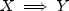(read, "if X, then Y"), where Y and X are disjoint frequent itemsets. The confidence measure associated with the rule is defined as the proportion of transactions containing X that also contain Y. Denote the support of X (the number of transactions containing X) as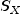and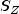is the support of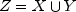. The confidence of the rule,is the ratio,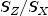. Note that the confidence ratio is the conditional probability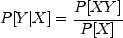where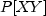denotes the probability of both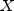and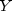. The probability of an itemsetis estimated by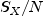, where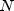is the total number of transactions.

Another measure of the strength of the association is known as the lift, which is the ratio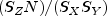. Lift values close to 1.0 suggest the sets are independent, and that they occur together by chance. Large lift values indicate a strong association. A minimum confidence threshold and a lift threshold can be specified.

If the transaction dataset is too large to fit in memory, Apriori can be applied to separate blocks of the transactions. The union of the frequent itemsets from each of the blocks becomes the set of candidate frequent itemsets. The frequencies of the itemsets are then accumulated over each block of data, and those with minimum support are the frequent itemsets for the entire dataset. The method is due to Savasere, Omiecinski, and Navathe (1995) and is also summarized and compared with other approaches in Rajaran and Ullman (2010).

Example 1, Example 2, Serialized Form
• ### Method Summary

Methods
Modifier and Type Method and Description
`static int[]` ```countFrequency(Itemsets candItemsets, int[][] x)```
Returns the frequency of each itemset in the transaction data set, `x`.
`static int[]` ```countFrequency(Itemsets candItemsets, int[][] x, int[] prevFrequencies)```
Returns the frequency of each itemset in the transaction data set, `x`, added to the previous frequencies.
`static AssociationRule[]` ```getAssociationRules(Itemsets itemsets, double confidence, double lift)```
Returns strong association rules among the itemsets in `itemsets`.
`static Itemsets` ```getFrequentItemsets(int[][] x, int maxNumProducts, int maxSetSize, double minPctSupport)```
Computes the frequent itemsets in the transaction set x.
`static Itemsets` ```getUnion(Itemsets itemsets1, Itemsets itemsets2)```
Return the union of two sets of itemsets.
`static int[]` ```sum(int[] freq1, int[] freq2)```
Sums up the itemset frequencies.
`static Itemsets` ```updateFrequentItemsets(Itemsets candItemsets, int[] freq)```
Updates the set of frequent items in `candItemsets`.
• ### Methods inherited from class java.lang.Object

`clone, equals, finalize, getClass, hashCode, notify, notifyAll, toString, wait, wait, wait`
• ### Method Detail

• #### countFrequency

```public static int[] countFrequency(Itemsets candItemsets,
int[][] x)```
Returns the frequency of each itemset in the transaction data set, `x`.
Parameters:
`candItemsets` - an `Itemsets` object containing the candidate itemsets and the corresponding number of transactions.
`x` - an `int[][]` containing the transaction data. The first column of `x` contains the transaction IDs, and the second column contains the item IDs. There will be a row for each transaction ID/ item ID combination, and all records for a single transaction ID must be in adjacent rows. Furthermore, the algorithm assumes that an individual transaction is complete within a single dataset. That is, there is no matching of transaction IDs between different data sets.
Returns:
an `int` array of length equal to the number of sets in `candItemsets` containing the frequencies of each itemset in `x`.
• #### countFrequency

```public static int[] countFrequency(Itemsets candItemsets,
int[][] x,
int[] prevFrequencies)```
Returns the frequency of each itemset in the transaction data set, `x`, added to the previous frequencies.
Parameters:
`candItemsets` - an `Itemsets` object containing the candidate itemsets and the corresponding number of transactions.
`x` - an `int[][]` containing the transaction data. The first column of `x` contains the transaction IDs, and the second column contains the item IDs. There will be a row for each transaction ID/ item ID combination, and all records for a single transaction ID must be in adjacent rows. Furthermore, the algorithm assumes that an individual transaction is complete within a single dataset. That is, there is no matching of transaction IDs between different data sets.
`prevFrequencies` - an `int` array containing the itemset frequencies counted so far in other blocks of transaction data.
Returns:
an `int` array of length equal to the number of sets in `candItemsets` containing the frequencies of each itemset in `x` added to `prevFrequencies`.
• #### getAssociationRules

```public static AssociationRule[] getAssociationRules(Itemsets itemsets,
double confidence,
double lift)```
Returns strong association rules among the itemsets in `itemsets`.

`confidence` and `lift` are the two criterion used to determine a strong association. If either is exceeded, the association rule will be considered "strong".

Parameters:
`itemsets` - an `Itemsets` object that contains the itemsets.
`confidence` - a `double` scalar containing the minimum confidence used to determine the strong association rules. `confidence` must be in [0,1].

`lift` is the other criterion which determines whether an association is "strong". If either criterion, `confidence` or `lift` is exceeded, the association rule will be considered "strong".

`lift` - a `double` scalar containing the minimum lift used to determine the strong association rules. `lift` must be non-negative.

If either criterion, `lift` or `confidence` is exceeded, the association rule will be considered "strong".

Returns:
an `AssociationRule` object containing association rules.
• #### getFrequentItemsets

```public static Itemsets getFrequentItemsets(int[][] x,
int maxNumProducts,
int maxSetSize,
double minPctSupport)```
Computes the frequent itemsets in the transaction set x.
Parameters:
`x` - an `int[][]` containing the transaction data. The first column of `x` contains the transaction IDs, and the second column contains the item IDs. There will be a row for each transaction ID/ item ID combination, and all records for a single transaction ID must be in adjacent rows. Furthermore, the algorithm assumes that an individual transaction is complete within a single dataset. That is, there is no matching of transaction IDs between different data sets.
`maxNumProducts` - an `int` scalar containing the maximum number of items or products that may be present in the transactions. `maxNumProducts` must be greater than or equal to the number of items in `x`.
`maxSetSize` - an `int` scalar containing the maximum size of an itemset.

Only frequent itemsets with `maxSetSize` or fewer items are considered in the analysis.

`minPctSupport` - a `double` scalar containing the minimum percentage of transactions in which an item or itemset must be present to be considered frequent. `minPctSupport` must be in the interval [0,1].
Returns:
an `Itemsets` containing the frequent itemsets.
• #### getUnion

```public static Itemsets getUnion(Itemsets itemsets1,
Itemsets itemsets2)```
Return the union of two sets of itemsets.

The attributes of each `Itemsets` object must agree. If `maxNumProducts`, `maxSetSize` or `minPctSupport` differ between the input arguments, an exception will be thrown.

Parameters:
`itemsets1` - an `Itemsets` object containing the frequent itemset for the union.
`itemsets2` - an `Itemsets` object containing the frequent itemset for the union.
Returns:
an `Itemsets` object containing the union of two itemsets.
• #### sum

```public static int[] sum(int[] freq1,
int[] freq2)```
Sums up the itemset frequencies.
Parameters:
`freq1` - an `Itemsets` object containing the itemset frequencies counted over one or more blocks of transaction data.
`freq2` - an `Itemsets` object containing the itemset frequencies counted over a second set of blocks of transaction data.
Returns:
an `int` array containing the sum of the frequencies.
• #### updateFrequentItemsets

```public static Itemsets updateFrequentItemsets(Itemsets candItemsets,
int[] freq)```
Updates the set of frequent items in `candItemsets`. The minimum support is determined by `minSupportPercentage` times the total number of transactions.
Parameters:
`candItemsets` - an `Itemsets` object containing the candidate itemsets and the corresponding number of transactions.
`freq` - an `int` array containing the frequencies for each itemset in `candItemsets`.
Returns:
an `Itemsets` object containing the updated itemsets.
JMSLTM Numerical Library 7.2.0